# 一文学懂递归和动态规划

09/21 08:37

## 前言

• 时空复杂度的详细分析
• 识别并简化递归过程中的重复运算
• 披上羊皮的狼
• 适当炫技助我拿到第一份工作

## 算法思路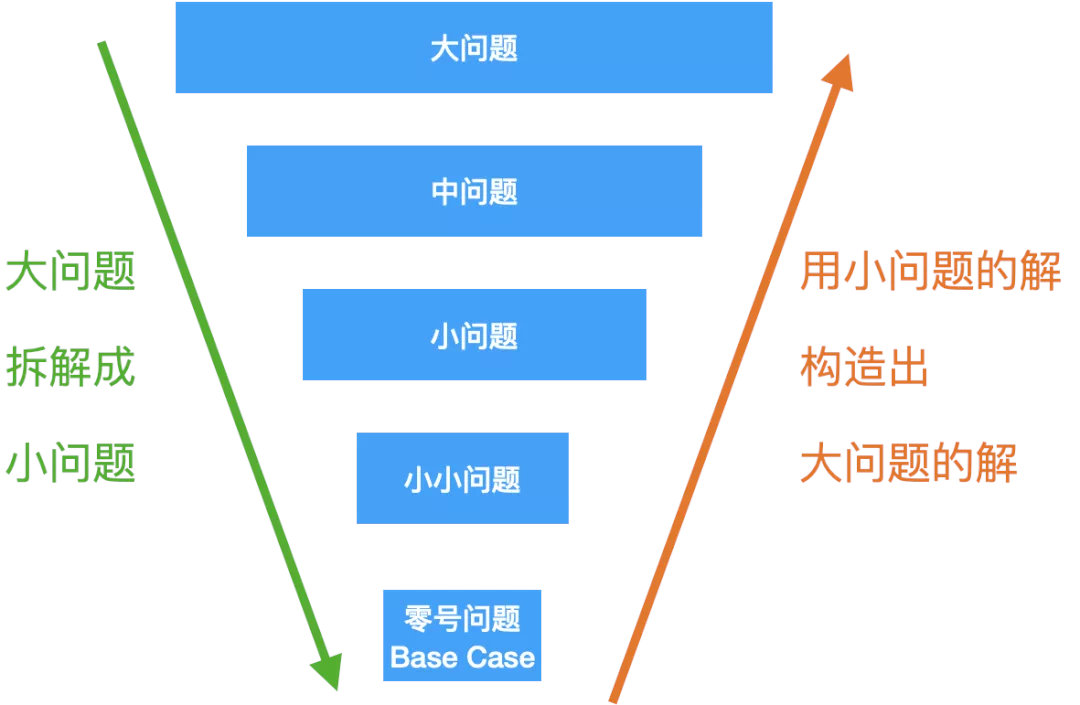Base case：就是递归的零号问题，也是递归的终点，走到最小的那个问题，能够直接给出结果，不必再往下走了，否则，就会成死循环；

## 斐波那契数列

### 解析

\$\$f(n) = f(n-1) + f(n-2)\$\$

• base case: f(0) = 0, f(1) = 1.
• 分解：f(n-1), f(n-2)
• 组合：f(n) = f(n-1) + f(n-2)

``````class Solution {
public int fib(int N) {
if (N == 0) {
return 0;
} else if (N == 1) {
return 1;
}
return fib(N-1) + fib(N-2);
}
}
``````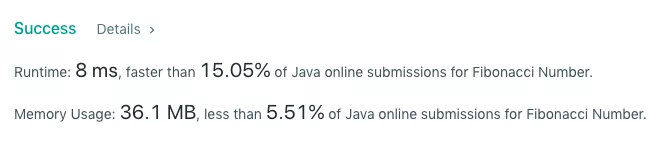### 过程分析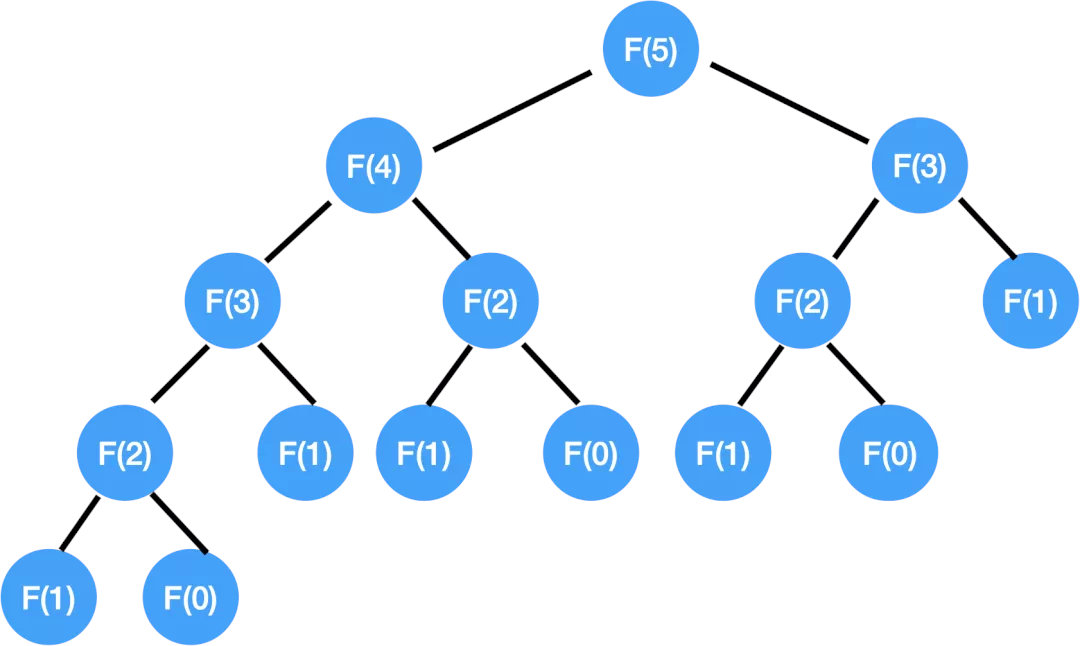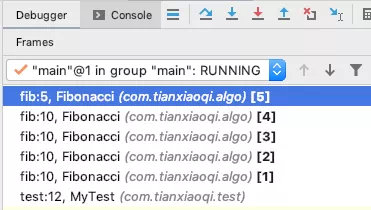<video src="../../2.%20%E5%85%AC%E4%BC%97%E5%8F%B7/5%20Recursion%20-%20Fib/%E5%8E%8B%E6%A0%88%E8%BF%87%E7%A8%8B%E8%AF%A6%E8%A7%A3.MP4"></video>

### 时间复杂度分析

Theta: 描述的是 tight bound
Omega(n): 这个描述的是 best case，最好的情况，没啥意义

<span style="color:blue">这也给我们了些许启发，不要说你平时表现有多好，没有意义；面试衡量的是你在 worst case 的水平；不要说面试没有发挥出你的真实水平，扎心的是那就是我们的真实水平。

`总时间 = 节点个数 * 每个节点的时间`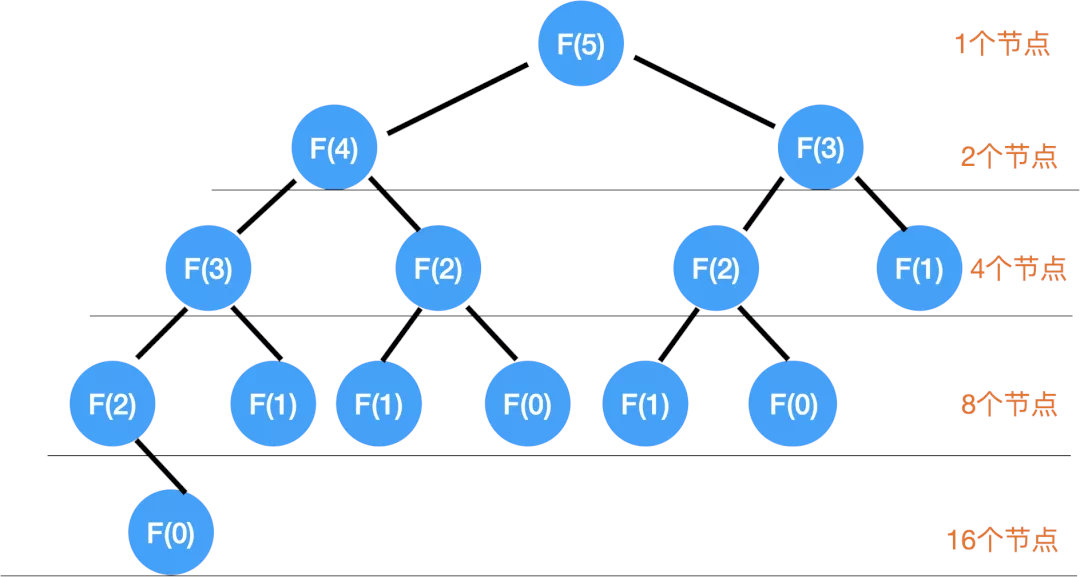`1 + 2 + 4 + 8 + 16`

<span style="color:blue"> 其实前面每一层的节点相加起来的个数都不会超过最后一层的节点的个数，总的节点数最多也就是最后一层节点数 * 2，然后在大 O 的时间复杂度里面常数项也是无所谓的，所以这个总的时间复杂度就是：

<span style="color:blue">

### 空间复杂度分析

`Auxiliary space complexity`

<span style="color:blue"> 举例说明区别：比如结果让你输出一个长度为 n 的数组，那么这 O(n) 的空间是不算在算法的空间复杂度里的，因为这个空间是跑不掉的，不是取决于你的算法的。

### 优化算法

`记录 F(0) ~ F(n-1) 的值`

Index 0 1 2 3 4 5
F(n) 0 1 1 2 3 5

``````class Solution {
public int fib(int N) {
if (N == 0) {
return 0;
}
if (N== 1) {
return 1;
}
int[] notes = new int[N+1];
notes = 0;
notes = 1;
for(int i = 2; i <= N; i++) {
notes[i] = notes[i-1] + notes[i-2];
}
return notes[N];
}
}
``````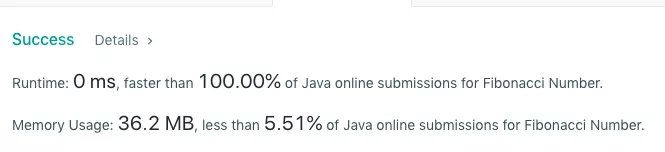``````class Solution {
public int fib(int N) {
int a = 0;
int b = 1;
if(N == 0) {
return a;
}
if(N == 1) {
return b;
}
for(int i = 2; i <= N; i++) {
int tmp = a + b;
a = b;
b = tmp;
}
return b;
}
}
``````

<span style="color:blue">那我们比较一下 Recursion 和 DP：

Recursion 是从大到小，层层分解，直到 base case 分解不了了再组合返回上去；
DP 是从小到大，记好笔记，不断进步。

## 披着羊皮的狼

<span style="color:blue">那有同学可能会想，这题这么简单，这都 2020 年了，面试还会考么？

``````f = lambda n: 1 if n in (1, 2) else f(n-1) + f(n-2)
``````

``````def fib(n)
a, b = 1, 1
for i in range(n-1):
a, b = b, a+b
return a
``````

<span style="color:blue">然后还写了个 caching 的方法:

``````def cache(f):
memo = {}
def helper(x):
if x not in memo:
memo[x] = f(x)
return memo[x]
return helper
@cache
def fibR(n):
if n==1 or n==2: return 1
return fibR(n-1) + fibR(n-2)
``````

<span style="color:blue">还顺便和面试官聊了下 tail recursion:

tail recursion 尾递归：就是递归的这句话是整个方法的最后一句话。

``````def fib(n, a=0, b=1):
if n==0: return a
if n==1: return b
return fib(n-1, b, a+b)
``````

<span style="color:blue">最终，拿出了我的杀手锏：lambda and reduce

``````fibRe = lambda n: reduce(lambda x, n: [x, x+x], range(n), [0, 1])
``````

## 前言

• 时空复杂度的详细分析
• 识别并简化递归过程中的重复运算
• 披上羊皮的狼
• 适当炫技助我拿到第一份工作

## 算法思路Base case：就是递归的零号问题，也是递归的终点，走到最小的那个问题，能够直接给出结果，不必再往下走了，否则，就会成死循环；

## 斐波那契数列

### 解析

\$\$f(n) = f(n-1) + f(n-2)\$\$

• base case: f(0) = 0, f(1) = 1.
• 分解：f(n-1), f(n-2)
• 组合：f(n) = f(n-1) + f(n-2)

``````class Solution {
public int fib(int N) {
if (N == 0) {
return 0;
} else if (N == 1) {
return 1;
}
return fib(N-1) + fib(N-2);
}
}
``````### 过程分析<video src="../../2.%20%E5%85%AC%E4%BC%97%E5%8F%B7/5%20Recursion%20-%20Fib/%E5%8E%8B%E6%A0%88%E8%BF%87%E7%A8%8B%E8%AF%A6%E8%A7%A3.MP4"></video>

### 时间复杂度分析

Theta: 描述的是 tight bound
Omega(n): 这个描述的是 best case，最好的情况，没啥意义

<span style="color:blue">这也给我们了些许启发，不要说你平时表现有多好，没有意义；面试衡量的是你在 worst case 的水平；不要说面试没有发挥出你的真实水平，扎心的是那就是我们的真实水平。

`总时间 = 节点个数 * 每个节点的时间``1 + 2 + 4 + 8 + 16`

<span style="color:blue"> 其实前面每一层的节点相加起来的个数都不会超过最后一层的节点的个数，总的节点数最多也就是最后一层节点数 * 2，然后在大 O 的时间复杂度里面常数项也是无所谓的，所以这个总的时间复杂度就是：

<span style="color:blue">

### 空间复杂度分析

`Auxiliary space complexity`

<span style="color:blue"> 举例说明区别：比如结果让你输出一个长度为 n 的数组，那么这 O(n) 的空间是不算在算法的空间复杂度里的，因为这个空间是跑不掉的，不是取决于你的算法的。

### 优化算法

`记录 F(0) ~ F(n-1) 的值`

Index 0 1 2 3 4 5
F(n) 0 1 1 2 3 5

``````class Solution {
public int fib(int N) {
if (N == 0) {
return 0;
}
if (N== 1) {
return 1;
}
int[] notes = new int[N+1];
notes = 0;
notes = 1;
for(int i = 2; i <= N; i++) {
notes[i] = notes[i-1] + notes[i-2];
}
return notes[N];
}
}
````````````class Solution {
public int fib(int N) {
int a = 0;
int b = 1;
if(N == 0) {
return a;
}
if(N == 1) {
return b;
}
for(int i = 2; i <= N; i++) {
int tmp = a + b;
a = b;
b = tmp;
}
return b;
}
}
``````

<span style="color:blue">那我们比较一下 Recursion 和 DP：

Recursion 是从大到小，层层分解，直到 base case 分解不了了再组合返回上去；
DP 是从小到大，记好笔记，不断进步。

## 披着羊皮的狼

<span style="color:blue">那有同学可能会想，这题这么简单，这都 2020 年了，面试还会考么？

``````f = lambda n: 1 if n in (1, 2) else f(n-1) + f(n-2)
``````

``````def fib(n)
a, b = 1, 1
for i in range(n-1):
a, b = b, a+b
return a
``````

<span style="color:blue">然后还写了个 caching 的方法:

``````def cache(f):
memo = {}
def helper(x):
if x not in memo:
memo[x] = f(x)
return memo[x]
return helper
@cache
def fibR(n):
if n==1 or n==2: return 1
return fibR(n-1) + fibR(n-2)
``````

<span style="color:blue">还顺便和面试官聊了下 tail recursion:

tail recursion 尾递归：就是递归的这句话是整个方法的最后一句话。

``````def fib(n, a=0, b=1):
if n==0: return a
if n==1: return b
return fib(n-1, b, a+b)
``````

<span style="color:blue">最终，拿出了我的杀手锏：lambda and reduce

``````fibRe = lambda n: reduce(lambda x, n: [x, x+x], range(n), [0, 1])
``````

1
7 收藏

### 作者的其它热门文章09/22 18:27

1 评论
7 收藏
1